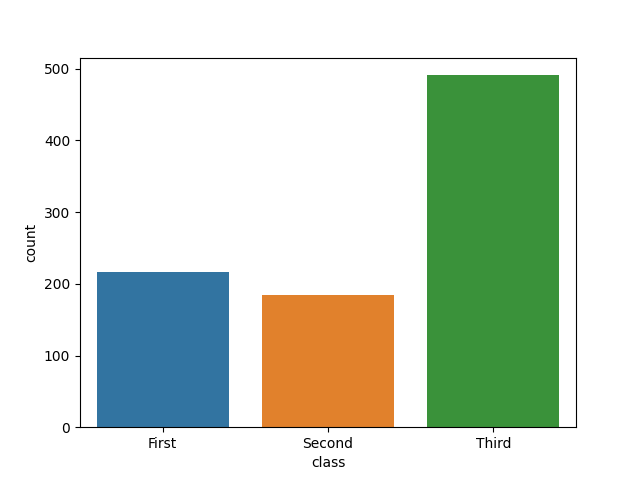Course Content

# Ultimate Visualization with Python

1. Matplotlib Introduction

2. Creating Commonly Used Plots

4. More Statistical Plots

5. Plotting with Seaborn

Ultimate Visualization with Python

##Countplot

A countplot is a plot which creates columns (bars) that represent the number of entries for each category of a categorical list. It can be also thought of as a histogram of a categorical variable. Let’s have a look at an example of a count plot:Here each column represents the number of Titanic passengers of each class. You may have already noticed that this plot is very similar to the bar chart. Indeed, it is a rather specific kind of bar chart representing the frequency of each category.

Note

You still have to import the `pyplot` module from `matplotlib` and use the `plt.show()` function to display the plots created with `seaborn`.

In order to create a countplot with `seaborn`, you should use the `countplot()` function. There are several possible options to pass our data to this function.

### Passing a 1D Array

The first option is to simply pass the value for the `x` parameter which can be some kind of an array:As you can see, the function simply counts the occurrences of each unique element in the list and creates a column with the respective height for each of them.

Note

We may also use y parameter instead of x to change the orientation of the plot from vertical to horizontal.

### Passing a 2D Object

Another option is to use the `data` parameter combined with the `x` or `y` parameter. This approach is suitable for working with `pandas` DataFrames. You can pass a list of arrays or a `DataFrame` as the value for `data`. For `x` or `y` you can pass a name of the column in the `DataFrame`, for example:Our function in this example creates a countplot based on the `'class'` column of a titanic `DataFrame` and counts the number of entries for each unique value in this column.

1. Import the `seaborn` library with the `sns` alias.
2. Import the `matplotlib.pyplot` module with the `plt` alias.
4. Use the `diamonds` as the first argument to specify the `DataFrame`.
5. Use the `'cut'` column of the `diamonds` DataFrame as the categories for the countplot and display the cateories on the y-axis via the second argument.